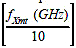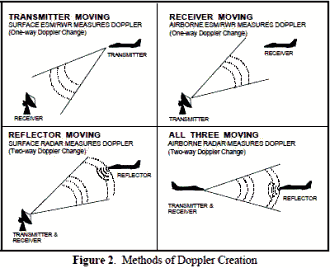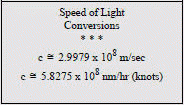About RF CafeCopyright: 1996 - 2024Webmaster:     BSEE - KB3UON RF Cafe began life in 1996 as "RF Tools" in an AOL screen name web space totaling 2 MB. Its primary purpose was to provide me with ready access to commonly needed formulas and reference material while performing my work as an RF system and circuit design engineer. The World Wide Web (Internet) was largely an unknown entity at the time and bandwidth was a scarce commodity. Dial-up modems blazed along at 14.4 kbps while typing up your telephone line, and a nice lady's voice announced "You've Got Mail" when a new message arrived... All trademarks, copyrights, patents, and other rights of ownership to images and text used on the RF Cafe website are hereby acknowledged. My Hobby Website: AirplanesAndRockets.com# Electronic Warfare and Radar Systems Engineering Handbook- Doppler Shift -

Doppler Shift

Doppler is the apparent change in wavelength (or frequency) of an electromagnetic or acoustic wave when there is relative movement between the transmitter (or frequency source) and the receiver.

 Summary RF Equation for the Two-Way (radar) caseSummary RF Equation for the One-Way (ESM) caseRules of Thumb for two-way signal travel (divide in half for one-way ESM signal measurements)At 10 GHz, fD ≈35 Hz per Knot 19 Hz per km/Hr67 Hz per m/sec61 Hz per yd/sec20 Hz per ft/sec

To estimate fD at other frequencies, multiply these by:The Doppler effect is shown in Figure 1. In everyday life this effect is commonly noticeable when a whistling train or police siren passes you. Audio Doppler is depicted, however Doppler can also affect the frequency of a radar carrier wave, the PRF of a pulse radar signal, or even light waves causing a shift of color to the observer.

How do we know the universe is expanding?

Answer: The color of light from distant stars is shifted to red (see Section 7-1: higher 8 or lower frequency means Doppler shift is stretched, i.e. expanding).

A memory aid might be that the lights from a car (going away) at night are red (tail lights)!Doppler frequency shift is directly proportional to velocity and a radar system can therefore be calibrated to measure velocity instead of (or along with) range. This is done by measuring the shift in frequency of a wave caused by an object in motion (Figure 2).

* Transmitter in motion

* Reflector in motion

* All three

For a closing relative velocity:

* Wave is compressed

* Frequency is increased

For an opening relative velocity:

* Wave is stretched

* Frequency is decreased

To compute Doppler frequency we note that velocity is range rate; V = dr/dtFor the reflector in motion case, You can see the wave compression effect in Figure 3 when the transmitted wave peaks are one wavelength apart. When the first peak reaches the target, they are still one wavelength apart (point a).

When the 2nd peak reaches the target, the target has advanced according to its velocity (vt) (point b), and the first reflected peak has traveled toward the radar by an amount that is less than the original wavelength by the same amount (vt) (point c).

As the 2nd peak is reflected, the wavelength of the reflected wave is 2(vt) less than the original wavelength (point d).

The distance the wave travels is twice the target range. The reflected phase lags transmitted phase by 2x the round trip time.

For a fixed target the received phase will differ from the transmitted phase by a constant phase shift. For a moving target the received phase will differ by a changing phase shift.

For the closing target shown in Figure 3, the received phase is advancing with respect to the transmitted phase and appears as a higher frequency.Doppler is dependent upon closing velocity, not actual radar or target velocity as shown in Figure 4.

For the following equations (except radar mapping), we assume the radar and target are moving directly toward one another in order to simplify calculations (if this is not the case, use the velocity component of one in the direction of the other in the formulas).

For the case of a moving reflector, Doppler frequency is proportional to 2x the transmitted frequency:

Higher rf = higher Doppler shift

fD = (2 x VTarget)(f/c)

Likewise, it can be shown that for other cases, the following relationships hold:For an airplane radar with an airplane target (The "all three moving" case)

For the case of a semi-active missile receiving signals (Also "all three moving")

fD = (VRadar + 2VTarget +VMissile)(f/c)

For the airplane radar with a ground target (radar mapping) or vice versa.

fD = 2(VRadar Cosθ Cosф)(f/c), Where 2 and N are the radar scan azimuth and depression angles.

For a ground based radar with airborne target - same as previous using target track crossing angle and ground radar elevation angle.

For the ES/ESM/RWR case where only the target or receiver is moving (One-way Doppler measurements)

fD = VReceiver or Target (f/c)

Note: See Figure 4 if radar and target are not moving directly towards or away from one another.Figure 5 depicts the results of a plot of the above equation for a moving reflector such as might be measured with a ground radar station illuminating a moving aircraft. It can be used for the aircraft-to-aircraft case, if the total net closing rate of the two aircraft is used for the speed entry in the figure. It can also be used for the ES/ESM case (one-way Doppler measurements) if the speed of the aircraft is used and the results are divided by two.

Sample Problems:

(1) If a ground radar operating at 10 GHz is tracking an airplane flying at a speed of 500 km/hr tangential to it (crossing pattern) at a distance of 10 km, what is the Doppler shift of the returning signal?

Answer: Since the closing velocity is zero, the Doppler is also zero.

(2) If the same aircraft turns directly toward the ground radar, what is the Doppler shift of the returning signal?

Answer: 500 km/hr = 270 kts from Section 2-1. From Figure 4 we see that the Doppler frequency is about 9.2 kHz.

(3) Given that a ground radar operating at 7 GHz is Doppler tracking an aircraft 20 km away (slant range) which is flying directly toward it at an altitude of 20,000 ft and a speed of 800 ft/sec, what amount of VGPO switch would be required of the aircraft jammer to deceive (pull) the radar to a zero Doppler return?

Answer: We use the second equation from the bottom of page 2-6.3 which is essentially the same for this application except a ground based radar is tracking an airplane target (versus an airplane during ground mapping), so for our application we use a positive elevation angle instead of a negative (depression) angle.

fD = 2(Vr Cos θ Cos ф)(f/c), where θ is the aircraft track crossing angle and ф is the radar elevation angle.

Since the aircraft is flying directly at the radar, 2 = θ°; the aircraft altitude = 20,000 ft = 6,096 meters.

Using the angle equation in Section 2-1, sin ф = x/r = altitude / slant range, so:

ф = sin-1 (altitude/slant range) = sin-1 (6,096 m / 20,000 m) = 17.7°

FD = 2(800 ft/sec Cos θ° Cos 17.7°)(7x10 Hz9 / 9.8357 x 109 ft/sec) = 10,845 HzPlease Support RF Cafe by purchasing my  ridiculously low−priced products, all of which I created. These Are Available for Free